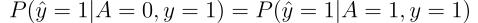[This article was first published on Stories by Przemyslaw Biecek on Medium, and kindly contributed to R-bloggers]. (You can report issue about the content on this page here)
Want to share your content on R-bloggers? click here if you have a blog, or here if you don't.

### fairmodels: let’s fight with biased Machine Learning models (part 1 — detection)

Author: Jakub Wiśniewski

### TL;DR

The fairmodels R Package facilitates bias detection through model visualizations. It implements few mitigation strategies that could reduce the bias. It enables easy to use checks for fairness metrics and comparison between different Machine Learning (ML) models.

### Longer version

Fairness in ML is a quickly emerging field. Big companies like IBM or Google developed some tools already (see AIF360) with growing community of users. Unfortunately, there aren’t many tools enabling to discover bias and discrimination in machine learning models created in R. Therefore, checking the fairness of the classifier created in R might be a difficult task. This is why R package fairmodels was created.

### Introduction to fairness concepts

What does it mean that model is fair? Imagine we have a classification model which decisions would have some impact on a human. For example, the model must decide whether some individuals will get a loan or not. What we don’t want is our model predictions to be based on sensitive (later called protected) attributes such as sex, race, nationality, etc… because it could potentially harm some unprivileged groups of people. However, not using such variables might not be enough because the correlations are usually hidden deep inside the data. That is what fairness in ML is for. It checks if privileged and unprivileged groups are treated similarly and if not, it offers some bias mitigation techniques.

There are numerous fairness metrics such as Statistical Parity, Equalized odds, Equal opportunity, and more. They check if model properties on privileged and unprivileged groups are the sameEqual opportunity criterion is satisfied when probabilities for 2 subgroups where A = 1 denotes privileged one are equal.

Many of these metrics can be derived from the confusion matrix. For example, Equal opportunity is ensuring the equal rate of TPR (True Positive Rate) among subgroups in the protected variable. However, knowing these rates is not essential information for us. We would like to know whether the difference between these rates between the privileged group and the unprivileged ones is significant. Let’s say that the acceptable difference in fairness metrics is 0.1. We will call this epsilon. TPR criterion for this metric would be:For all subgroups (unique values in the protected variable) the fairness metric difference between subgroup denoted as i and the privileged one must be lower than some acceptable value epsilon ( 0.1 in our case ).

Such a criterion is double-sided. It also ensures that there is not much difference in favour of the unprivileged group.

### fairmodels as bias detection tool

fairmodels is R package for discovering, eliminating, and visualizing bias. Its main function — fairness_check() enables the user to quickly check if popular fairness metrics are satisfied. fairness_check() return an object called fairness_object. It wraps models together with metrics in useful structure. To create this object we need to provide:

• Classification model explained with DALEX
• The protected variable in the form of a factor. Unlike in other fairness tools, it doesn’t need to be binary, just discrete.
• The privileged group in the form of character or factor

So let’s see how it works in practice. We will make a linear regression model with german credit data predicting whether a certain person makes more or less than 50k annually. Sex will be used as a protected variable.

1. Create a model
```library(fairmodels)
data("german")
y_numeric <- as.numeric(german\$Risk) -1
lm_model <- glm(Risk~., data = german, family=binomial(link="logit"))```

2. Create an explainer

```library(DALEX)
explainer_lm <- explain(lm_model, data = german[,-1], y = y_numeric)```

3. Use the fairness_check(). Here the epsilon value is set to default which is 0.1

```fobject <- fairness_check(explainer_lm,
protected = german\$Sex,
privileged = "male")```

Now we can check the level of biasprint(fobject)- prints information in console. Tells us how many metrics model passes and what is the total difference (loss) in all metricsplot(object) — returns ggplot object. Shows red and green areas, where the red field signifies bias. If the bar reaches the left red field it means that the unprivileged group is discriminated, if it reaches the right red zone it means that there is a bias towards the privileged group.

As we can see checking fairness is not difficult. What is more complicated is comparing the discrimination between models. But even this can be easily done with fairmodels!

### fairmodels is flexible

When we have many models, they can be passed into one fairness_check() together. Moreover, there is possible an iterative approach. As we explain the model and it does not satisfy fairness criteria, we can add other models along with fairness_object to fairness_check(). That way even the same model with different parameters and/or trained on different data can be compared with the previous one(s).

```library(ranger)
rf_model     <- ranger(Risk ~., data = german, probability = TRUE)
explainer_rf <- explain(rf_model, data = german[,-1], y = y_numeric)
fobject      <- fairness_check(explainer_rf, fobject)```

That is it. Ranger model passes our fairness criteria (epsilon = 0.1) and therefore is fair.

### Summary

fairmodels is flexible and easy to use tool for asserting that the ML model is fair. It can handle multiple models, trained on different data no matter if it was encoded, features were standardized, etc… It facilitates the bias detection process in multiple models allowing at the same time to compare those models with each other.